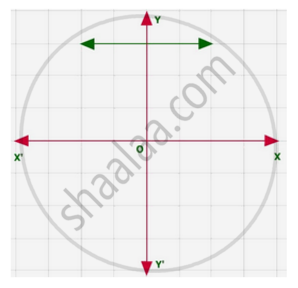# The graphs of y = p(x) are given in following figure, for some polynomials p(x). Find the number of zeroes of p(x), in each case - Mathematics

Sum

The graphs of y = p(x) are given in following figure, for some polynomials p(x). Find the number of zeroes of p(x), in the following.#### Solution

The graph does not intersects at x-axis.

∴ The no of zeroes of p(x) is 0

Concept: Geometrical Meaning of the Zeroes of a Polynomial
Is there an error in this question or solution?

#### APPEARS IN

NCERT Class 10 Maths
Chapter 2 Polynomials
Exercise 2.1 | Q 1.1 | Page 28